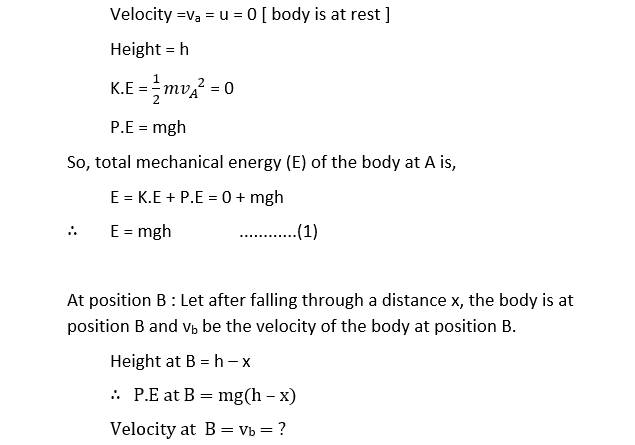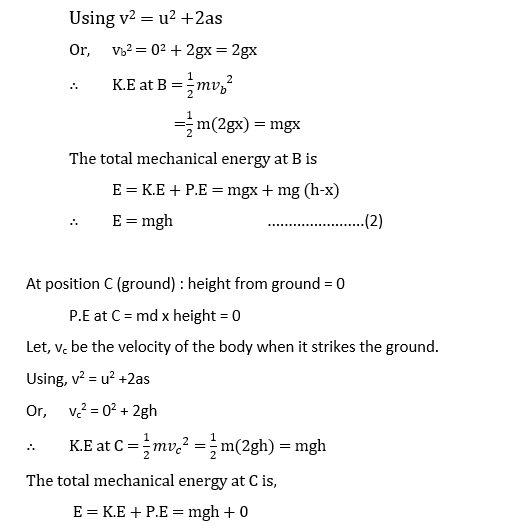# Principle of Conservation of EnergyPrinciple of conservation of energy states that, “energy can neither be created nor be destroyed but can be converted from one form to another form.” Therefore, the total energy of the universe is constant.

Principle of conservation of the Mechanical Energy

When a body falls freely from a height , its kinetic energy goes on increasing. The gain in kinetic energy is at the expense of the gravitational potential energy, which is reduced. However, total mechanical energy of the body remains conserved at each and every points of it’s path.

Suppose a body having mass ‘m’ is falling freely as shown in figure:Let ‘h’ be the height of the body above the reference level (ground) at initial position A.

At position A:∴         E = mgh                                        .............................(3)

From equations (1),(2) and (3) it is clear that the total mechanical energy (sum of K.E and P.E) of the freely falling body remains constant at all stages of its motion .

The graph shows that the variation of K.E and P.E with the height from ground(reference level: